| 首页 |

#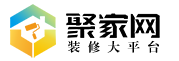A B C D E F G H J K L M N P Q R S T W X Y Z

• A

• B

• C

• D

• E

• F

• G

• H

• J

• K

• L

• M

• N

• P

• Q

• R

• S

• T

• W

• X

• Y

• Z

A B C D E F G H J K L M N P Q R S T W X Y Z

• A

• B

• C

• D

• E

• F

• G

• H

• J

• K

• L

• M

• N

• P

• Q

• R

• S

• T

• W

• X

• Y

• Z

# 免费申请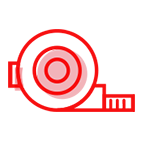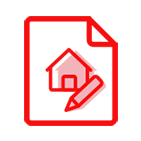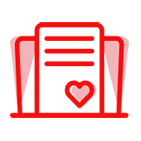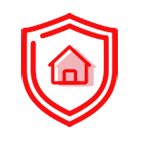14738

53256010001

181

×

×

#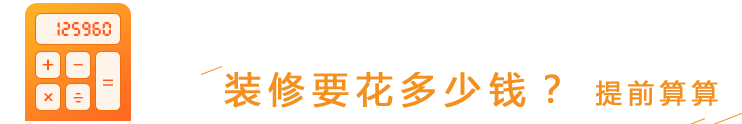### 装修计算器

* 请选择所在地

* 请输入正确的房屋面积

* 请输入正确的联系方式

• 材料费： ?
• 人工费： ?
• 设计费： ?
• 质检费： ?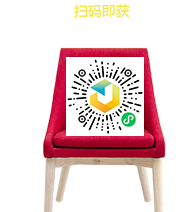• 材料费： 10000
• 人工费： 10000
• 设计费： 0 11300元
• 质检费： 0 11300元

* 报价有疑问？稍后装修管家将致电为您解答

* 该报价为毛坯半包价，实际装修报价以量房实测为准### 完善以下信息   让我们更了解您的需求优先为您服务

1. 您家的房屋现状是 :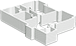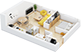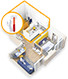2. 您家准备什么时候开始装修 :

3. 您家小区名称 :